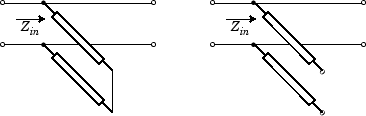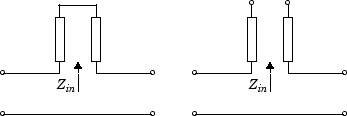rfckt.coaxial

Coaxial transmission line

Description

Use the coaxial class to represent coaxial transmission lines that are characterized by line dimensions, stub type, and termination.

A coaxial transmission line is shown in cross-section in the following figure. Its physical characteristics include the radius of the inner conductor of the coaxial transmission line a, and the radius of the outer conductor b.Creation

Description

example

h = rfckt.coaxial returns a coaxial transmission line object whose properties are set to their default values.

h = rfckt.coaxial(Name,Value) sets properties using one or more name-value pairs. For example, rfckt.coaxial('OuterRadius',0.0043) creates a coaxial transmission line object with outer radius of 0.0043 meters. You can specify multiple name-value pairs. Enclose each property name in quotes. Properties not specified retain their default values.

Properties

expand all

Computed S-parameters, noise figure, OIP3, and group delay values, specified as an rfdata.data object. This is a read-only property. For more information refer, Algorithms.

Data Types: function_handle

Relative permittivity of dielectric, specified as a scalar. The relative permittivity is the ratio of permittivity of the dielectric,$\epsilon$, to the permittivity in free space, ${\epsilon }_{0}$. The default value is2.3.

Data Types: double

Inner conductor radius, specified as a scalar in meters. The default value is 7.25e-4.

Data Types: double

Physical length of transmission line, specified as a scalar in meters. The default value is 0.01.

Data Types: double

Tangent of loss angle of dielectric, specified as a scalar. The default value is 0.

Data Types: double

Relative permeability of dielectric, specified as a scalar. The ratio of permeability of dielectric, $\mu$, to the permeability in free space, ${\mu }_{0}$. The default value is 1.

Data Types: double

Object name, specified as a 1-by-N character array.

Data Types: char

Number of ports, specified as a positive integer.

Data Types: double

Outer conductor radius, specified as a scalar in meters. The default value is 0.0026.

Data Types: double

Conductor conductivity, specified as a scalar in Siemens per meter (S/m). The default value is Inf.

Data Types: double

Type of stub, specified as one of the following values: 'NotaStub', 'Series', 'Shunt'.

Data Types: double

Stub transmission line termination, specified as one of the following values: 'NotApplicable', 'Open', 'Short'.

Data Types: double

Object Functions

 analyze Analyze RFCKT object in frequency domain calculate Calculate specified parameters for rfckt objects or rfdata objects circle Draw circles on Smith Chart extract Extract specified network parameters from rfckt object or data object listformat List valid formats for specified circuit object parameter listparam List valid parameters for specified circuit object loglog Plot specified circuit object parameters using log-log scale plot Plot circuit object parameters on X-Y plane plotyy Plot parameters of RF circuit or RF data on X-Y plane with two Y-axes getop Display operating conditions polar Plot specified object parameters on polar coordinates semilogx Plot RF circuit object parameters using log scale for x-axis semilogy Plot RF circuit object parameters using log scale for y-axis smith Plot circuit object parameters on Smith chart write Write RF data from circuit or data object to file getz0 Calculate characteristic impedance of RFCKT transmission line object read Read RF data from file to new or existing circuit or data object restore Restore data to original frequencies getop Display operating conditions

Examples

collapse all

Create a coaxial transmission line with 0.0045 meters outer radius using rfckt.coaxial.

tx1 =
rfckt.coaxial with properties:

MuR: 1
EpsilonR: 2.3000
LossTangent: 0
SigmaCond: Inf
LineLength: 0.0100
StubMode: 'NotAStub'
Termination: 'NotApplicable'
nPort: 2
AnalyzedResult: []
Name: 'Coaxial Transmission Line'

Algorithms

The analyze method treats the transmission line as a 2-port linear network. It computes the AnalyzedResult property of a stub or as a stubless line using the data stored in the rfckt.coaxial object properties as follows:

• If you model the transmission line as a stubless line, the analyze method first calculates the ABCD-parameters at each frequency contained in the modeling frequencies vector. It then uses the abcd2s function to convert the ABCD-parameters to S-parameters.

The analyze method calculates the ABCD-parameters using the physical length of the transmission line, d, and the complex propagation constant, k, using the following equations:

$\begin{array}{l}A=\frac{{e}^{kd}+{e}^{-kd}}{2}\\ B=\frac{{Z}_{0}*\left({e}^{kd}-{e}^{-kd}\right)}{2}\\ C=\frac{{e}^{kd}-{e}^{-kd}}{2*{Z}_{0}}\\ D=\frac{{e}^{kd}+{e}^{-kd}}{2}\end{array}$

Z0 and k are vectors whose elements correspond to the elements of f, the vector of frequencies specified in the analyze input argument freq. Both can be expressed in terms of the resistance (R), inductance (L), conductance (G), and capacitance (C) per unit length (meters) as follows:

$\begin{array}{c}{Z}_{0}=\sqrt{\frac{R+j2\pi fL}{G+j2\pi fC}}\\ k={k}_{r}+j{k}_{i}=\sqrt{\left(R+j2\pi fL\right)\left(G+j2\pi FC\right)}\end{array}$

where

$\begin{array}{c}R=\frac{1}{2\pi {\sigma }_{cond}{\delta }_{cond}}\left(\frac{1}{a}+\frac{1}{b}\right)\\ L=\frac{\mu }{2\pi }\mathrm{ln}\left(\frac{b}{a}\right)\\ G=\frac{2\pi \omega {\epsilon }^{″}}{\mathrm{ln}\left(\frac{b}{a}\right)}\\ C=\frac{2\pi \epsilon }{\mathrm{ln}\left(\frac{b}{a}\right)}\end{array}$

In these equations:

• a is the radius of the inner conductor.

• b is the radius of the outer conductor.

• σcond is the conductivity in the conductor.

• μ is the permeability of the dielectric.

• ε is the permittivity of the dielectric.

• ε″ is the imaginary part of ε, ε″  = ε0εrtan δ, where:

• ε0 is the permittivity of free space.

• εr is the EpsilonR property value.

• tan δ is the LossTangent property value.

• δcond is the skin depth of the conductor, which the method calculates as $1/\sqrt{\pi f\mu {\sigma }_{cond}}$.

• f is a vector of modeling frequencies determined by the Outport (RF Blockset) block.

• If you model the transmission line as a shunt or series stub, the analyze method first calculates the ABCD-parameters at the specified frequencies. It then uses the abcd2s function to convert the ABCD-parameters to S-parameters.

When you set the StubMode property to 'Shunt', the 2-port network consists of a stub transmission line that you can terminate with either a short circuit or an open circuit as shown in the following figure.Zin is the input impedance of the shunt circuit. The ABCD-parameters for the shunt stub are calculated as:

$\begin{array}{c}A=1\\ B=0\\ C=1/{Z}_{in}\\ D=1\end{array}$

When you set the StubMode property to 'Series', the 2-port network consists of a series transmission line that you can terminate with either a short circuit or an open circuit as shown in the following figure.Zin is the input impedance of the series circuit. The ABCD-parameters for the series stub are calculated as

$\begin{array}{c}A=1\\ B={Z}_{in}\\ C=0\\ D=1\end{array}$

 Pozar, David M. Microwave Engineering, John Wiley & Sons, Inc., 2005.